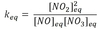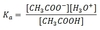# Equilibrium Flashcards Preview

## Chemistry 101 > Equilibrium > Flashcards

Flashcards in Equilibrium Deck (70):
1

## the law of mass action

### The law of mass action states that, at equilibrium, the composition of the reaction mixture can be expressed in terms of an ideal equilibrium constant, keq. For the chemical reaction aA (g) + bB (g) ⇔ cC (g) if [C]eq is the concentration of C at equilibrium,2

## For the chemical reaction aA (g) + bB (g) ⇔ cC (g) what is the value of the reaction quotient Q?

### The expression for Q is very similar to the expression for keq. The only difference is that Q can apply to a chemical system at any concentrations, while keq specifically refers to the concentrations at equilibrium.3

4

## What is the equilibrium constant formula for the reaction: NO + NO3 ⇔ 2NO2

### The value of the equilibrium constant is calculated from the actual concentrations of the products and reactants at equilibrium. The general formula for this reaction is:5

6

7

8

9

10

11

## aA (g) + bB (g) ⇔ cC (g) is in equilibrium, and additional A is added, what happens to the reaction quotient Q?12

13

14

15

16

17

18

19

20

21

22

23

24

25

26

27

28

29

30

31

32

33

34

35

36

37

38

39

40

41

42

43

## Ka

### Ka is the acid dissociation constant for an acid in solution. The higher the Ka the stronger the acid. Ex: for acetic acid, CH3COOH, Ka can be calculated as:44

## What is the Ka equation for the reaction of acetic acid and water? CH3COOH + H2O ⇒ CH3COO- + H3O+

### Remember: Keq equations include only components in the gaseous or aqueous phase; components in the solid or liquid phase, like H2O, are ignored.45

46

47

48

49

50

51

52

53

54

55

56

57

58

59

60

61

62

63

64

65

66

67

68

69

70